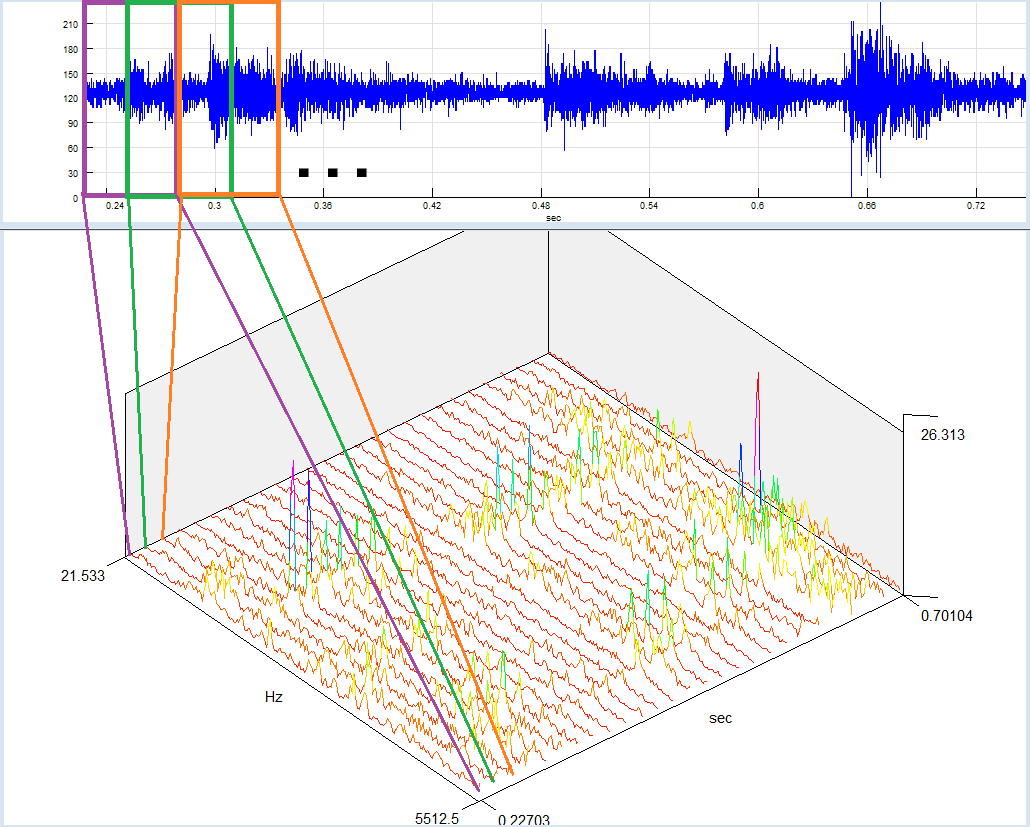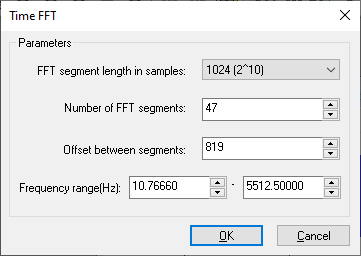The Time-FFT function calculates change of signals frequency components through time. The signal is divided in smaller, equally spaced segments (possibly overlapped), an FFT for each segment is calculated, and all FFT sequences are used to build a 3D graphic. The same calculation method is used for Spectrogram view.The FFT calculation parameters depend on your current Spectral analysis defaults. You can change the parameters for the calculated Time-FFT at any time by selecting the “Edit/Properties” option from the main menu or “Properties” from the context menu.

By selecting the “3D-Tools/Time-FFT” option from the main menu, you can set Time-FFT parameters, calculate it, and display as a 3D graphic.

Time FFT parametersFFT segment length in samples: Length of a signal segment for the FFT calculation.

Number of FFT segments: Number of signal segments. This can be calculated from the segment length and offset parameters.

Offset between segments: Distance (in samples) between beginnings of consecutive signal segments. This can be calculated from FFT segment length and the number of FFT segments.

Frequency range: Frequency range you would like to see in your graphics.

Spectrogram

A special case of Time-FFT analysis is the Spectrogram this is calculated in the same way as Time-FFT, except the 3D-graphic is shown from above and coloured so that the amplitude differences are color-coded (same amplitudes always has the same color). Selecting the “3D tools/Spectrogram” option from the main menu brings up the same dialog as in the case of Time-FFT, except that resulting graphics are shown as a spectrogram.

The time axis is always shown from left to right (X-axis). The direction of the frequency axis (Y-axis) can be changed by using the Application settings dialog.

Editing Time-FFT/Spectrogram properties

After creating a spectrogram or Time-FFT window, you can change its parameters (FFT length, number of segments, etc.) by selecting the "Time-FFT properties..." option from its context menu.

There is an even faster way to change properties of the Time-FFT/Spectrogram window by using a Ctrl + mouse wheel:

Moving it to the front (up) will increase time resolution and decrease frequency resolution by a factor of 2

Moving it back (down) will decrease time resolution and increase frequency resolution by a factor of 2